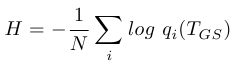A supertagger works in a similar way to a part-of-speech tagger. It assigns a category or set of categories to each element in the observation sequence. My supertagger models assign a probability distribution over possible categories to each observation. In the normal use of a supertagger, a certain beam will be set so that only the most probable categories are used by the parser.

The models are trained on annotated sequences from my corpus. I evaluate the taggers using cross-validation and compare the tag distribution returned to the gold standard tags from the corpus.

On this page, I describe some measures that I use to evaluate different supertagging models against one another.

Tags or categories

In the context of supertagging, I use the terms tag and category interchangeably. The taggers assign tags to observations, but each tag represents a category in the grammar.

# Top Tag Accuracy

The simplest measure of the accuracy of the tagger is to take just the most probable tag predicted by the tagger and check whether this is the tag in the gold standard. This emulates what the supertagger would do if it were used with a very harsh beam and allowed to choose only one tag for each chord.

The figure reported is simply the proportion of tags returned that are identical to the gold standard.

# N-Best Tag Accuracy

For some value of N, we can report the N-best tag accuracy of the tagger. This is like the top tag accuracy, but allows the gold standard tag to be found among the N highest probability tags. This will always be highest than the top tag accuracy (which is this measure with N=1).

For some small value of N (maybe 3 or 4), this is a more realistic approximation to the actual performance of the tagger, since a tagger will usually be asked for more than one possible tag by the parser. In practice, the number of tags required will depend on the parses the parser gets (it may request more tags if it can't parse using the initial set), and possibly also on the relative probabilities of the tags.

The figure is the proportion of gold standard tags that were found among the highest probability N tags according to the model's output.

# Cross Entropy

A more revealing measure is the cross entropy between the gold standard tag distribution and the distribution given by the tagging model. The gold standard distribution is taken to be that which gives the gold standard tag a probability of 1 and all others 0.

In effect this means that the figure is the arithmetic mean of the negative log probabilities assigned by the model to the gold standard tags. A lower value means that the correct tags were on average given a higher probability, so lower is better.

The cross entropy of probability distributions p and q is defined as:

•However, the probability of the tag in the gold standard distribution is 0 for all tags other than the gold standard tag, so this value is simply the negative log probability assigned by the model to the gold standard tag.

We then average this over all the chords in all the sequences of the test set. This gives us the cross entropy per chord.

•The downside of this measure is that it is a lot less intuitive than the accuracy measure.

The benefit is that it distinguishes between two models that both pick the wrong tag for a chord, but where one gives the right one a higher probability.